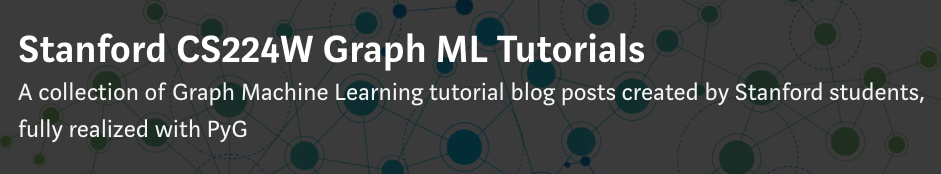# Colab Notebooks and Video Tutorials

We have prepared a list of colab notebooks that practically introduces you to the world of Graph Neural Networks with PyG:

Stanford CS224W Graph ML Tutorials:The Stanford CS224W course has collected a set of graph machine learning tutorial blog posts, fully realized with PyG. Students worked on projects spanning all kinds of tasks, model architectures and applications. All tutorials also link to a Google Colab with the code in the tutorial for you to follow along with as you read it!

PyTorch Geometric Tutorial Project:

The PyTorch Geometric Tutorial project provides video tutorials and Colab notebooks for a variety of different methods in PyG:

1. Introduction [Video, Notebook]

2. PyTorch basics [Video, Notebook]

3. Graph Attention Networks (GATs) [Video, Notebook]

4. Spectral Graph Convolutional Layers [Video, Notebook]

5. Aggregation Functions in GNNs [Video, Notebook]

6. (Variational) Graph Autoencoders (GAE and VGAE) [Video, Notebook]

7. Adversarially Regularized Graph Autoencoders (ARGA and ARGVA) [Video, Notebook]

8. Graph Generation [Video]

9. Recurrent Graph Neural Networks [Video, Notebook (Part 1), Notebook (Part 2)]

10. DeepWalk and Node2Vec [Video (Theory), Video (Practice), Notebook]

11. Edge analysis [Video, Notebook (Link Prediction), Notebook (Label Prediction)]

12. Data handling in PyG (Part 1) [Video, Notebook]

13. Data handling in PyG (Part 2) [Video, Notebook]

14. MetaPath2vec [Video, Notebook]

15. Graph pooling (DiffPool) [Video, Notebook]